## Autocorrelation

The autocorrelation function is defined by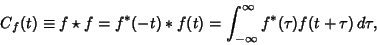(1)

where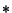denotes Convolution and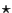denotes Cross-Correlation. A finite autocorrelation is given by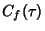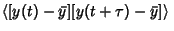(2)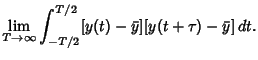(3)

If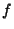is a Real Function,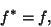(4)

and an Even Function so that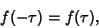(5)

then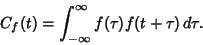(6)

But let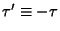, so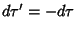, then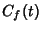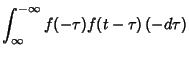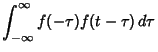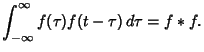(7)

The autocorrelation discards phase information, returning only the Power. It is therefore not reversible.

There is also a somewhat surprising and extremely important relationship between the autocorrelation and the Fourier Transform known as the Wiener-Khintchine Theorem. Let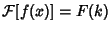, and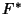denote the Complex Conjugate of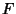, then the Fourier Transform of the Absolute Square of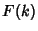is given by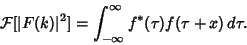(8)

The autocorrelation is a Hermitian Operator since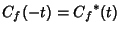.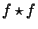is Maximum at the Origin. In other words,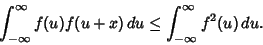(9)

To see this, let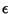be a Real Number. Then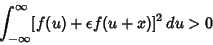(10)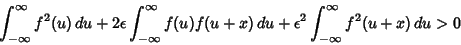(11)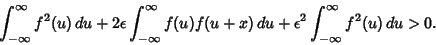(12)

Define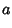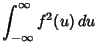(13)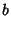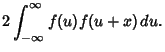(14)

Then plugging into above, we have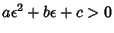. This Quadratic Equation does not have any Real Root, so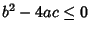, i.e.,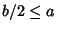. It follows that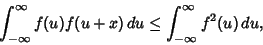(15)

with the equality at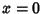. This proves thatis Maximum at the Origin.

Press, W. H.; Flannery, B. P.; Teukolsky, S. A.; and Vetterling, W. T. Correlation and Autocorrelation Using the FFT.'' §13.2 in Numerical Recipes in FORTRAN: The Art of Scientific Computing, 2nd ed. Cambridge, England: Cambridge University Press, pp. 538-539, 1992.